Do you like the article?
Share it with others —
Use new possibilities of MetaTrader 5

#### Similar articles# MQL5 Cookbook: How to Avoid Errors When Setting/Modifying Trade Levels

MetaTrader 5Examples | 16 April 2013, 13:2112 03210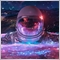### Introduction

In continuation of our work on the Expert Advisor from the previous article of the series called "MQL5 Cookbook: Analyzing Position Properties in the MetaTrader 5 Strategy Tester", we will enhance it with a whole lot of useful functions, as well as improve and optimize the existing ones.

Questions from beginners regarding errors arising when setting/modifying trade levels (Stop Loss, Take Profit and pending orders) are far from being rare in MQL programming forum(s). I believe many of you must already be familiar with the journal message ending with [Invalid stops]. In this article, we will create functions that normalize and check trade level values for correctness before opening/modifying a position.

The Expert Advisor will this time have external parameters that can be optimized in the MetaTrader 5 Strategy Tester and will in some ways resemble a simple trading system. We certainly still have a long way to go before we can develop a real trading system. But Rome wasn't built in a day. So we have yet a lot to do.

Code optimization in the existing functions will be considered as the article unfolds. The info panel will not be dealt with at this point as we still need to have a look at some position properties that cannot be obtained using standard identifiers (use of history of deals is required). This subject will nevertheless be covered in one of the following articles of the series.

Let us start. As usual, we begin with inserting additional enumerations, variables, arrays and auxiliary functions at the beginning of the file. We will need a function that will allow us to easily get symbol properties. The same simple approach will be necessary in getting position properties.

We saw in the previous articles that global variables were assigned all position properties at once in the GetPositionProperties function. This time, we will try to provide a possibility of getting each property separately. Below are two enumerations for the implementation of the above. The functions themselves will be reviewed a little later.

```//--- Enumeration of position properties
enum ENUM_POSITION_PROPERTIES
{
P_SYMBOL        = 0,
P_MAGIC         = 1,
P_COMMENT       = 2,
P_SWAP          = 3,
P_COMMISSION    = 4,
P_PRICE_OPEN    = 5,
P_PRICE_CURRENT = 6,
P_PROFIT        = 7,
P_VOLUME        = 8,
P_SL            = 9,
P_TP            = 10,
P_TIME          = 11,
P_ID            = 12,
P_TYPE          = 13,
P_ALL           = 14
};
//--- Enumeration of symbol properties
enum ENUM_SYMBOL_PROPERTIES
{
S_DIGITS       = 0,
S_STOPSLEVEL   = 2,
S_POINT        = 3,
S_BID          = 5,
S_VOLUME_MIN   = 6,
S_VOLUME_MAX   = 7,
S_VOLUME_LIMIT = 8,
S_VOLUME_STEP  = 9,
S_FILTER       = 10,
S_UP_LEVEL     = 11,
S_DOWN_LEVEL   = 12,
S_ALL          = 13
};```

The ENUM_SYMBOL_PROPERTIES enumeration does not contain all symbol properties but they can be added at any time, as necessary. The enumeration also contains user-defined properties (10, 11, 12) whose calculation is based on other symbol properties. There is an identifier that can be used to get all properties from the enumeration at once, like in the enumeration of position properties.

This is followed by the external parameters of the Expert Advisor:

```//--- External parameters of the Expert Advisor
input int            NumberOfBars=2;     // Number of Bullish/Bearish bars for a Buy/Sell
input double         Lot         =0.1;   // Lot
input double         StopLoss    =50;    // Stop Loss
input double         TakeProfit  =100;   // Take Profit
input double         TrailingStop=10;    // Trailing Stop
input bool           Reverse     =true;  // Position reverse```

Let us have a closer look at the external parameters:

• NumberOfBars - this parameter sets the number of one direction bars for opening a position;
• Lot - position volume;
• TakeProfit - Take Profit level in points. Zero value means that no Take Profit needs to be set.
• StopLoss - Stop Loss level in points. Zero value means that no Stop Loss needs to be set.
• TrailingStop - Trailing Stop value in points. For a BUY position, the calculation is based on the bar's minimum (minimum minus the number of points from the StopLoss parameter). For a SELL position, the calculation is based on the bar's maximum (maximum plus the number of points from the StopLoss parameter). Zero value denotes that the Trailing Stop is off.
• Reverse enables/disables reverse position.

It is only the NumberOfBars parameter that needs further clarification. There is no point in setting this parameter value to, for example, more than 5 as this is quite rare and it would already be late to open a position after such movement. We will therefore need a variable that will help us adjust the value of this parameter:

```//--- To check the value of the NumberOfBars external parameter
int                  AllowedNumberOfBars=0;```

This parameter will also determine the amount of bar data that will be stored in price arrays. This will be discussed in a while when we get to modify the custom functions.

As in the case with position properties, we declare variables in global scope for symbol properties in order to provide access from any function:

```//--- Symbol properties
int                  sym_digits=0;           // Number of decimal places
int                  sym_stops_level=0;      // Stops level
double               sym_point=0.0;          // Point value
double               sym_bid=0.0;            // Bid price
double               sym_volume_min=0.0;     // Minimum volume for a deal
double               sym_volume_max=0.0;     // Maximum volume for a deal
double               sym_volume_limit=0.0;   // Maximum permissible volume for a position and orders in one direction
double               sym_volume_step=0.0;    // Minimum volume change step for a deal
double               sym_offset=0.0;         // Offset from the maximum possible price for a transaction
double               sym_up_level=0.0;       // Upper Stop level price
double               sym_down_level=0.0;     // Lower Stop level price```

Since the Trailing Stop must be calculated based on the bar's high and low, we will need arrays for such bar data:

```//--- Price data arrays
double               close_price[]; // Close (closing prices of the bar)
double               open_price[];  // Open (opening prices of the bar)
double               high_price[];  // High (bar's highs)
double               low_price[];   // Low (bar's lows)```

Let us now proceed to modifying and creating functions. We already have the GetBarsData function that copies opening and closing prices of bars to price arrays. Now, we also need highs and lows. In addition, the value obtained from the NumberOfBars parameter should be adjusted. This is what the function looks like after the modification:

```//+------------------------------------------------------------------+
//| Getting bar values                                               |
//+------------------------------------------------------------------+
void GetBarsData()
{
//--- Adjust the number of bars for the position opening condition
if(NumberOfBars<=1)
AllowedNumberOfBars=2;              // At least two bars are required
if(NumberOfBars>=5)
AllowedNumberOfBars=5;              // but no more than 5
else
AllowedNumberOfBars=NumberOfBars+1; // and always more by one
//--- Reverse the indexing order (... 3 2 1 0)
ArraySetAsSeries(close_price,true);
ArraySetAsSeries(open_price,true);
ArraySetAsSeries(high_price,true);
ArraySetAsSeries(low_price,true);
//--- Get the closing price of the bar
//    If the number of the obtained values is less than requested, print the relevant message
if(CopyClose(_Symbol,Period(),0,AllowedNumberOfBars,close_price)<AllowedNumberOfBars)
{
Print("Failed to copy the values ("
+_Symbol+", "+TimeframeToString(Period())+") to the Close price array! "
"Error "+IntegerToString(GetLastError())+": "+ErrorDescription(GetLastError()));
}
//--- Get the opening price of the bar
//    If the number of the obtained values is less than requested, print the relevant message
if(CopyOpen(_Symbol,Period(),0,AllowedNumberOfBars,open_price)<AllowedNumberOfBars)
{
Print("Failed to copy the values ("
+_Symbol+", "+TimeframeToString(Period())+") to the Open price array! "
"Error "+IntegerToString(GetLastError())+": "+ErrorDescription(GetLastError()));
}
//--- Get the bar's high
//    If the number of the obtained values is less than requested, print the relevant message
if(CopyHigh(_Symbol,Period(),0,AllowedNumberOfBars,high_price)<AllowedNumberOfBars)
{
Print("Failed to copy the values ("
+_Symbol+", "+TimeframeToString(Period())+") to the High price array! "
"Error "+IntegerToString(GetLastError())+": "+ErrorDescription(GetLastError()));
}
//--- Get the bar's low
//    If the number of the obtained values is less than requested, print the relevant message
if(CopyLow(_Symbol,Period(),0,AllowedNumberOfBars,low_price)<AllowedNumberOfBars)
{
Print("Failed to copy the values ("
+_Symbol+", "+TimeframeToString(Period())+") to the Low price array! "
"Error "+IntegerToString(GetLastError())+": "+ErrorDescription(GetLastError()));
}
}```

The conditions requiring at least two bars and always more by one are there because we will only go by completed bars that start with index . In fact, adjustments can in this case be treated as unnecessary as the bar data can be copied starting from the index specified in the third parameter of the CopyOpen, CopyClose, CopyHigh and CopyLow functions. The limit of 5 bars can also be changed (upward/downward) at your own discretion.

The GetTradingSignal function has now become a little more complex as the condition will be generated differently depending on the number of bars specified in the NumberOfBars parameter. Further, we now use a more correct type of the returned value - order type:

```//+------------------------------------------------------------------+
//+------------------------------------------------------------------+
{
if(AllowedNumberOfBars==2 &&
close_price>open_price)
if(AllowedNumberOfBars==3 &&
close_price>open_price &&
close_price>open_price)
if(AllowedNumberOfBars==4 &&
close_price>open_price &&
close_price>open_price &&
close_price>open_price)
if(AllowedNumberOfBars==5 &&
close_price>open_price &&
close_price>open_price &&
close_price>open_price &&
close_price>open_price)
if(AllowedNumberOfBars>=6 &&
close_price>open_price &&
close_price>open_price &&
close_price>open_price &&
close_price>open_price &&
close_price>open_price)
//--- A Sell signal (ORDER_TYPE_SELL) :
if(AllowedNumberOfBars==2 &&
close_price<open_price)
return(ORDER_TYPE_SELL);
if(AllowedNumberOfBars==3 &&
close_price<open_price &&
close_price<open_price)
return(ORDER_TYPE_SELL);
if(AllowedNumberOfBars==4 &&
close_price<open_price &&
close_price<open_price &&
close_price<open_price)
return(ORDER_TYPE_SELL);
if(AllowedNumberOfBars==5 &&
close_price<open_price &&
close_price<open_price &&
close_price<open_price &&
close_price<open_price)
return(ORDER_TYPE_SELL);
if(AllowedNumberOfBars>=6 &&
close_price<open_price &&
close_price<open_price &&
close_price<open_price &&
close_price<open_price &&
close_price<open_price)
return(ORDER_TYPE_SELL);
//--- No signal (WRONG_VALUE):
return(WRONG_VALUE);
}```

Let us now modify the GetPositionProperties function. In the previous articles, it allowed us to get all properties at once. However, sometimes you may only need to get one property. To do this, you can certainly use the standard functions offered by the language, yet this would not be as convenient as we want. Below is the code of the GetPositionProperties function modified. Now, when passing a certain identifier from the ENUM_POSITION_PROPERTIES enumeration, you can either get a certain single position property or all the properties at once.

```//+------------------------------------------------------------------+
//| Getting position properties                                      |
//+------------------------------------------------------------------+
void GetPositionProperties(ENUM_POSITION_PROPERTIES position_property)
{
//--- Check if there is an open position
pos_open=PositionSelect(_Symbol);
//--- If an open position exists, get its properties
if(pos_open)
{
switch(position_property)
{
case P_SYMBOL        : pos_symbol=PositionGetString(POSITION_SYMBOL);                  break;
case P_MAGIC         : pos_magic=PositionGetInteger(POSITION_MAGIC);                   break;
case P_COMMENT       : pos_comment=PositionGetString(POSITION_COMMENT);                break;
case P_SWAP          : pos_swap=PositionGetDouble(POSITION_SWAP);                      break;
case P_COMMISSION    : pos_commission=PositionGetDouble(POSITION_COMMISSION);          break;
case P_PRICE_OPEN    : pos_price=PositionGetDouble(POSITION_PRICE_OPEN);               break;
case P_PRICE_CURRENT : pos_cprice=PositionGetDouble(POSITION_PRICE_CURRENT);           break;
case P_PROFIT        : pos_profit=PositionGetDouble(POSITION_PROFIT);                  break;
case P_VOLUME        : pos_volume=PositionGetDouble(POSITION_VOLUME);                  break;
case P_SL            : pos_sl=PositionGetDouble(POSITION_SL);                          break;
case P_TP            : pos_tp=PositionGetDouble(POSITION_TP);                          break;
case P_TIME          : pos_time=(datetime)PositionGetInteger(POSITION_TIME);           break;
case P_ID            : pos_id=PositionGetInteger(POSITION_IDENTIFIER);                 break;
case P_TYPE          : pos_type=(ENUM_POSITION_TYPE)PositionGetInteger(POSITION_TYPE); break;
case P_ALL           :
pos_symbol=PositionGetString(POSITION_SYMBOL);
pos_magic=PositionGetInteger(POSITION_MAGIC);
pos_comment=PositionGetString(POSITION_COMMENT);
pos_swap=PositionGetDouble(POSITION_SWAP);
pos_commission=PositionGetDouble(POSITION_COMMISSION);
pos_price=PositionGetDouble(POSITION_PRICE_OPEN);
pos_cprice=PositionGetDouble(POSITION_PRICE_CURRENT);
pos_profit=PositionGetDouble(POSITION_PROFIT);
pos_volume=PositionGetDouble(POSITION_VOLUME);
pos_sl=PositionGetDouble(POSITION_SL);
pos_tp=PositionGetDouble(POSITION_TP);
pos_time=(datetime)PositionGetInteger(POSITION_TIME);
pos_id=PositionGetInteger(POSITION_IDENTIFIER);
pos_type=(ENUM_POSITION_TYPE)PositionGetInteger(POSITION_TYPE);                     break;
default: Print("The passed position property is not listed in the enumeration!");               return;
}
}
//--- If there is no open position, zero out variables for position properties
else
ZeroPositionProperties();
}```

Similarly, we implement the GetSymbolProperties function for getting symbol properties:

```//+------------------------------------------------------------------+
//| Getting symbol properties                                        |
//+------------------------------------------------------------------+
void GetSymbolProperties(ENUM_SYMBOL_PROPERTIES symbol_property)
{
int lot_offset=1; // Number of points for the offset from the Stops level
//---
switch(symbol_property)
{
case S_DIGITS        : sym_digits=(int)SymbolInfoInteger(_Symbol,SYMBOL_DIGITS);                   break;
case S_POINT         : sym_point=SymbolInfoDouble(_Symbol,SYMBOL_POINT);                           break;
//---
sym_digits=(int)SymbolInfoInteger(_Symbol,SYMBOL_DIGITS);
case S_BID           :
sym_digits=(int)SymbolInfoInteger(_Symbol,SYMBOL_DIGITS);
sym_bid=NormalizeDouble(SymbolInfoDouble(_Symbol,SYMBOL_BID),sym_digits);                       break;
//---
case S_VOLUME_MIN    : sym_volume_min=SymbolInfoDouble(_Symbol,SYMBOL_VOLUME_MIN);                 break;
case S_VOLUME_MAX    : sym_volume_max=SymbolInfoDouble(_Symbol,SYMBOL_VOLUME_MAX);                 break;
case S_VOLUME_LIMIT  : sym_volume_limit=SymbolInfoDouble(_Symbol,SYMBOL_VOLUME_LIMIT);             break;
case S_VOLUME_STEP   : sym_volume_step=SymbolInfoDouble(_Symbol,SYMBOL_VOLUME_STEP);               break;
//---
case S_FILTER        :
sym_digits=(int)SymbolInfoInteger(_Symbol,SYMBOL_DIGITS);
sym_point=SymbolInfoDouble(_Symbol,SYMBOL_POINT);
sym_offset=NormalizeDouble(CorrectValueBySymbolDigits(lot_offset*sym_point),sym_digits);        break;
//---
case S_UP_LEVEL      :
sym_digits=(int)SymbolInfoInteger(_Symbol,SYMBOL_DIGITS);
sym_point=SymbolInfoDouble(_Symbol,SYMBOL_POINT);
//---
case S_DOWN_LEVEL    :
sym_digits=(int)SymbolInfoInteger(_Symbol,SYMBOL_DIGITS);
sym_point=SymbolInfoDouble(_Symbol,SYMBOL_POINT);
sym_bid=NormalizeDouble(SymbolInfoDouble(_Symbol,SYMBOL_BID),sym_digits);
sym_down_level=NormalizeDouble(sym_bid-sym_stops_level*sym_point,sym_digits);                   break;
//---
case S_ALL           :
sym_digits=(int)SymbolInfoInteger(_Symbol,SYMBOL_DIGITS);
sym_point=SymbolInfoDouble(_Symbol,SYMBOL_POINT);
sym_bid=NormalizeDouble(SymbolInfoDouble(_Symbol,SYMBOL_BID),sym_digits);
sym_volume_min=SymbolInfoDouble(_Symbol,SYMBOL_VOLUME_MIN);
sym_volume_max=SymbolInfoDouble(_Symbol,SYMBOL_VOLUME_MAX);
sym_volume_limit=SymbolInfoDouble(_Symbol,SYMBOL_VOLUME_LIMIT);
sym_volume_step=SymbolInfoDouble(_Symbol,SYMBOL_VOLUME_STEP);
sym_offset=NormalizeDouble(CorrectValueBySymbolDigits(lot_offset*sym_point),sym_digits);
sym_down_level=NormalizeDouble(sym_bid-sym_stops_level*sym_point,sym_digits);                   break;
//---
default: Print("The passed symbol property is not listed in the enumeration!"); return;
}
}```

Please note that some symbol properties may require you to get other properties first.

We have a new function, CorrectValueBySymbolDigits. It returns the relevant value, depending on the number of decimal places in the price. An integer or a real number can be passed to the function. The type of the passed data determines the version of the function to be used. This feature is called function overloading.

```//+------------------------------------------------------------------+
//| Adjusting the value based on the number of digits in the price (int)|
//+------------------------------------------------------------------+
int CorrectValueBySymbolDigits(int value)
{
return (sym_digits==3 || sym_digits==5) ? value*=10 : value;
}
//+------------------------------------------------------------------+
//| Adjusting the value based on the number of digits in the price (double)|
//+------------------------------------------------------------------+
double CorrectValueBySymbolDigits(double value)
{
return (sym_digits==3 || sym_digits==5) ? value*=10 : value;
}```

Our Expert Advisor will have an external parameter for specifying the volume (Lot) of the opening position. Let us create a function that will adjust the lot according to the symbol specification - CalculateLot:

```//+------------------------------------------------------------------+
//| Calculating position lot                                         |
//+------------------------------------------------------------------+
double CalculateLot(double lot)
{
//--- To adjust as per the step
double corrected_lot=0.0;
//---
GetSymbolProperties(S_VOLUME_MIN);  // Get the minimum possible lot
GetSymbolProperties(S_VOLUME_MAX);  // Get the maximum possible lot
GetSymbolProperties(S_VOLUME_STEP); // Get the lot increase/decrease step
//--- Adjust as per the lot step
corrected_lot=MathRound(lot/sym_volume_step)*sym_volume_step;
//--- If less than the minimum, return the minimum
if(corrected_lot<sym_volume_min)
return(NormalizeDouble(sym_volume_min,2));
//--- If greater than the maximum, return the maximum
if(corrected_lot>sym_volume_max)
return(NormalizeDouble(sym_volume_max,2));
//---
return(NormalizeDouble(corrected_lot,2));
}```

Let us now proceed to functions that are directly relevant to the title of the article. They are quite simple and straightforward and you can understand their purpose without any difficulty using the comments in the code.

The CalculateTakeProfit function is used to calculate the Take Profit value:

```//+------------------------------------------------------------------+
//| Calculating the Take Profit value                                |
//+------------------------------------------------------------------+
double CalculateTakeProfit(ENUM_ORDER_TYPE order_type)
{
//--- If Take Profit is required
if(TakeProfit>0)
{
//--- For the calculated Take Profit value
double tp=0.0;
//--- If you need to calculate the value for a SELL position
if(order_type==ORDER_TYPE_SELL)
{
//--- Calculate the level
tp=NormalizeDouble(sym_bid-CorrectValueBySymbolDigits(TakeProfit*sym_point),sym_digits);
//--- Return the calculated value if it is lower than the lower limit of the Stops level
//    If the value is higher or equal, return the adjusted value
return(tp<sym_down_level ? tp : sym_down_level-sym_offset);
}
//--- If you need to calculate the value for a BUY position
{
//--- Calculate the level
//--- Return the calculated value if it is higher that the upper limit of the Stops level
//    If the value is lower or equal, return the adjusted value
return(tp>sym_up_level ? tp : sym_up_level+sym_offset);
}
}
//---
return(0.0);
}```

The CalculateStopLoss function is used to calculate the Stop Loss value:

```//+------------------------------------------------------------------+
//| Calculating the Stop Loss value                                  |
//+------------------------------------------------------------------+
double CalculateStopLoss(ENUM_ORDER_TYPE order_type)
{
//--- If Stop Loss is required
if(StopLoss>0)
{
//--- For the calculated Stop Loss value
double sl=0.0;
//--- If you need to calculate the value for a BUY position
{
// Calculate the level
//--- Return the calculated value if it is lower that the lower limit of the Stops level
//    If the value is higher or equal, return the adjusted value
return(sl<sym_down_level ? sl : sym_down_level-sym_offset);
}
//--- If you need to calculate the value for a SELL position
if(order_type==ORDER_TYPE_SELL)
{
//--- Calculate the level
sl=NormalizeDouble(sym_bid+CorrectValueBySymbolDigits(StopLoss*sym_point),sym_digits);
//--- Return the calculated value if it is higher than the upper limit of the Stops level
//    If the value is lower or equal, return the adjusted value
return(sl>sym_up_level ? sl : sym_up_level+sym_offset);
}
}
//---
return(0.0);
}```

The CalculateTrailingStop function is used to calculate the Trailing Stop value:

```//+------------------------------------------------------------------+
//| Calculating the Trailing Stop value                              |
//+------------------------------------------------------------------+
double CalculateTrailingStop(ENUM_POSITION_TYPE position_type)
{
//--- Variables for calculations
double            level       =0.0;
double            sell_point  =high_price;   // The High value for a Sell
//--- Calculate the level for a BUY position
{
//--- Bar's low minus the specified number of points
//--- If the calculated level is lower than the lower limit of the Stops level,
//    the calculation is complete, return the current value of the level
if(level<sym_down_level)
return(level);
//--- If it is not lower, try to calculate based on the bid price
else
{
level=NormalizeDouble(sym_bid-CorrectValueBySymbolDigits(StopLoss*sym_point),sym_digits);
//--- If the calculated level is lower than the limit, return the current value of the level
//    otherwise set the nearest possible value
return(level<sym_down_level ? level : sym_down_level-sym_offset);
}
}
//--- Calculate the level for a SELL position
if(position_type==POSITION_TYPE_SELL)
{
// Bar's high plus the specified number of points
level=NormalizeDouble(sell_point+CorrectValueBySymbolDigits(StopLoss*sym_point),sym_digits);
//--- If the calculated level is higher than the upper limit of the Stops level,
//    the calculation is complete, return the current value of the level
if(level>sym_up_level)
return(level);
//--- If it is not higher, try to calculate based on the ask price
else
{
//--- If the calculated level is higher than the limit, return the current value of the level
//    Otherwise set the nearest possible value
return(level>sym_up_level ? level : sym_up_level+sym_offset);
}
}
//---
return(0.0);
}```

Now we have all the necessary functions that return correct values for trading operations. Let us create a function that will check a condition for modifying the Trailing Stop and will modify the same, if the specified condition is met - ModifyTrailingStop. Below is the code of this function with detailed comments.

Please pay attention to the use of all the functions created/modified above. The switch switch determines the relevant condition depending on the type of the current position and the condition result is then stored in the condition variable. To modify a position, we use the PositionModify method from the CTrade class of the Standard Library.

```//+------------------------------------------------------------------+
//| Modifying the Trailing Stop level                                |
//+------------------------------------------------------------------+
void ModifyTrailingStop()
{
//--- If the Trailing Stop and Stop Loss are set
if(TrailingStop>0 && StopLoss>0)
{
double         new_sl=0.0;       // For calculating the new Stop Loss level
bool           condition=false;  // For checking the modification condition
//--- Get the flag of presence/absence of the position
pos_open=PositionSelect(_Symbol);
//--- If the position exists
if(pos_open)
{
//--- Get the symbol properties
GetSymbolProperties(S_ALL);
//--- Get the position properties
GetPositionProperties(P_ALL);
//--- Get the Stop Loss level
new_sl=CalculateTrailingStop(pos_type);
//--- Depending on the position type, check the relevant condition for the Trailing Stop modification
switch(pos_type)
{
//--- If the new Stop Loss value is higher
//    than the current value plus the set step
condition=new_sl>pos_sl+CorrectValueBySymbolDigits(TrailingStop*sym_point);
break;
case POSITION_TYPE_SELL :
//--- If the new Stop Loss value is lower
//    than the current value minus the set step
condition=new_sl<pos_sl-CorrectValueBySymbolDigits(TrailingStop*sym_point);
break;
}
//--- If there is a Stop Loss, compare the values before modification
if(pos_sl>0)
{
//--- If the condition for the order modification is met, i.e. the new value is lower/higher
//    than the current one, modify the Trailing Stop of the position
if(condition)
{
Print("Error modifying the position: ",GetLastError()," - ",ErrorDescription(GetLastError()));
}
}
//--- If there is no Stop Loss, simply set it
if(pos_sl==0)
{
Print("Error modifying the position: ",GetLastError()," - ",ErrorDescription(GetLastError()));
}
}
}
}```

Now let us adjust the TradingBlock function in accordance with all the above changes. Just like in the ModifyTrailingStop function, all values of variables for a trading order will be determined using the switch switch. It significantly reduces the amount of code and simplifies further modifications since instead of one branch for two position types, only one remains.

```//+------------------------------------------------------------------+
//+------------------------------------------------------------------+
{
ENUM_ORDER_TYPE      signal=WRONG_VALUE;                 // Variable for getting a signal
string               comment="hello :)";                 // Position comment
double               tp=0.0;                             // Take Profit
double               sl=0.0;                             // Stop Loss
double               lot=0.0;                            // Volume for position calculation in case of reverse position
double               position_open_price=0.0;            // Position opening price
ENUM_ORDER_TYPE      order_type=WRONG_VALUE;             // Order type for opening a position
ENUM_POSITION_TYPE   opposite_position_type=WRONG_VALUE; // Opposite position type
//--- Get a signal
//--- If there is no signal, exit
if(signal==WRONG_VALUE)
return;
//--- Find out if there is a position
pos_open=PositionSelect(_Symbol);
//--- Get all symbol properties
GetSymbolProperties(S_ALL);
//--- Determine values for trade variables
switch(signal)
{
//--- Assign values to variables for a BUY
opposite_position_type=POSITION_TYPE_SELL;
break;
//--- Assign values to variables for a SELL
case ORDER_TYPE_SELL :
position_open_price=sym_bid;
order_type=ORDER_TYPE_SELL;
break;
}
//--- Calculate the Take Profit and Stop Loss levels
sl=CalculateStopLoss(order_type);
tp=CalculateTakeProfit(order_type);
//--- If there is no position
if(!pos_open)
{
lot=CalculateLot(Lot);
//--- Open a position
//    If the position failed to open, print the relevant message
{
Print("Error opening the position: ",GetLastError()," - ",ErrorDescription(GetLastError()));
}
}
//--- If there is a position
else
{
//--- Get the position type
GetPositionProperties(P_TYPE);
//--- If the position is opposite to the signal and the position reverse is enabled
if(pos_type==opposite_position_type && Reverse)
{
//--- Get the position volume
GetPositionProperties(P_VOLUME);
lot=pos_volume+CalculateLot(Lot);
//--- Open the position. If the position failed to open, print the relevant message
{
Print("Error opening the position: ",GetLastError()," - ",ErrorDescription(GetLastError()));
}
}
}
//---
return;
}```

We also need to make another important correction in the SetInfoPanel function but let us first prepare a few auxiliary functions that indicate how/where the program is currently used:

```//+------------------------------------------------------------------+
//| Returning the testing flag                                       |
//+------------------------------------------------------------------+
bool IsTester()
{
return(MQL5InfoInteger(MQL5_TESTER));
}
//+------------------------------------------------------------------+
//| Returning the optimization flag                                  |
//+------------------------------------------------------------------+
bool IsOptimization()
{
return(MQL5InfoInteger(MQL5_OPTIMIZATION));
}
//+------------------------------------------------------------------+
//| Returning the visual testing mode flag                           |
//+------------------------------------------------------------------+
bool IsVisualMode()
{
return(MQL5InfoInteger(MQL5_VISUAL_MODE));
}
//+------------------------------------------------------------------+
//| Returning the flag for real time mode outside the Strategy Tester|
//| if all conditions are met                                        |
//+------------------------------------------------------------------+
bool IsRealtime()
{
if(!IsTester() && !IsOptimization() && !IsVisualMode())
return(true);
else
return(false);
}```

The only thing we need to add to the SetInfoPanel function is a condition indicating to the program that the info panel should only be displayed in the visualization and real time modes. If this is ignored, the testing time will be 4-5 times longer. This is especially important when optimizing the parameters.

```//+------------------------------------------------------------------+
//| Setting the info panel                                           |
//|------------------------------------------------------------------+
void SetInfoPanel()
{
//--- Visualization or real time modes
if(IsVisualMode() || IsRealtime())
{
// The remaining code of the SetInfoPanel() function
// ...
}
}```

Now, we just need to make some changes to the main program functions to be able to proceed to the parameter optimization and Expert Advisor testing.

```//+------------------------------------------------------------------+
//| Expert initialization function                                   |
//+------------------------------------------------------------------+
int OnInit()
{
//--- Initialize the new bar
CheckNewBar();
//--- Get the properties and set the panel
GetPositionProperties(P_ALL);
//--- Set the info panel
SetInfoPanel();
//---
return(0);
}```
```//+------------------------------------------------------------------+
//| Expert tick function                                             |
//+------------------------------------------------------------------+
void OnTick()
{
//--- If the bar is not new, exit
if(!CheckNewBar())
return;
//--- If there is a new bar
else
{
GetBarsData();          // Get bar data
ModifyTrailingStop();   // Modify the Trailing Stop level
}
//--- Get the properties and update the values on the panel
GetPositionProperties(P_ALL);
//--- Update the info panel
SetInfoPanel();
}```
```//+------------------------------------------------------------------+
//+------------------------------------------------------------------+
{
//--- Get position properties and update the values on the panel
GetPositionProperties(P_ALL);
//--- Update the info panel
SetInfoPanel();
}```

### Optimizing Parameters and Testing Expert Advisor

Let us now optimize the parameters. We will make the Strategy Tester settings as shown below: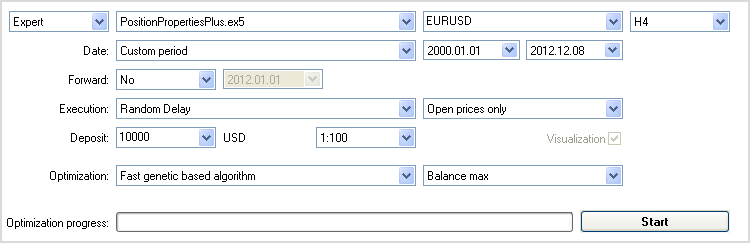Fig. 1. Strategy Tester settings for parameter optimization.

The Expert Advisor parameters will be given a wide range of values: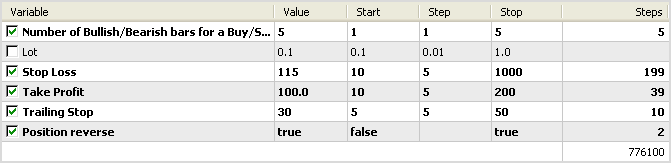Fig. 2. Expert Advisor settings for parameter optimization.

The optimization took about 7 minutes on a dual-core processor (Intel Core2 Duo P7350 @ 2.00GHz). Maximum recovery factor test results are as follows: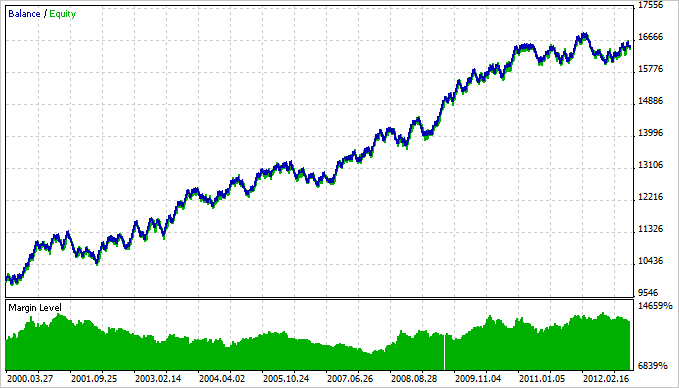Fig. 3. Maximum recovery factor test results.

### Conclusion

That's about it for now. Study, test, optimize, experiment and wow. The source code of the Expert Advisor featured in the article can be downloaded using the below link for further studying.

Translated from Russian by MetaQuotes Ltd.
Original article: https://www.mql5.com/ru/articles/643

Attached files |

#### Other articles by this author

Last comments | Go to discussion (10)| 22 Oct 2014 at 21:05
marketeer:
I'm afraid we need some code in order to help you. Most probable thing is that the error does exist in your code. Check that your stops are larger than minimal allowed distance and that you did not, by any chance, misplace buy and sell stops. Also make sure your broker does allow specifing stops at position opening - some of them require to add stops only to existing positions (so called "market execution").

Hi I tested only expert advisor attached to the article How to avoid errors when setting/modifying trade level, without any change (Positionpropertiesplus). Instatrader platform by Instaforex. The functions in this advisor are written so, that if i set wrong stops parameters they are set to lowest allowed. So normaly should i obtain no error prompt - that is true by forex pairs. But by GOLD in this platform I obtain by each attempt of positionpropertiesplus EA to open position with stops, the error prompt invalid stops and no position in strategy tester was open. Is that the case you mentioned - problem with instatrader platform and broker? In GOLD symbol properties is written instant execution.

Here is example of function for stoploss calculation from mentioned EA - I used the EA attached to the mentioned article without any change, i only used  GOLD instead of forex pairs:

```//+------------------------------------------------------------------+

//| Calculating the Stop Loss value                                  |

//+------------------------------------------------------------------+

double CalculateStopLoss(ENUM_ORDER_TYPE order_type)

{

//--- If Stop Loss is required

if(StopLoss>0)

{

//--- For the calculated Stop Loss value

double sl=0.0;

//--- If you need to calculate the value for a BUY position

{

// Calculate the level

//--- Return the calculated value if it is lower than the lower limit of the Stops level

//    If the value is higher or equal, return the adjusted value

return(sl<sym_down_level ? sl : sym_down_level-sym_offset);

}

//--- If you need to calculate the value for a SELL position

if(order_type==ORDER_TYPE_SELL)

{

//--- Calculate the level

sl=NormalizeDouble(sym_bid+CorrectValueBySymbolDigits(StopLoss*sym_point),sym_digits);

//--- Return the calculated value if it is higher that the upper limit of the Stops level

//    If the value is lower or equal, return the adjusted value

return(sl>sym_up_level ? sl : sym_up_level+sym_offset);

}

}

//---

return(0.0);

} ```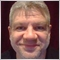| 25 Oct 2014 at 12:36
...

Hello,

Please use the SRC button when you post code. Thank you.

This time, I edited it for you.| 28 Oct 2014 at 21:18

Hi I tested only expert advisor attached to the article How to avoid errors when setting/modifying trade level, without any change (Positionpropertiesplus). Instatrader platform by Instaforex. The functions in this advisor are written so, that if i set wrong stops parameters they are set to lowest allowed. So normaly should i obtain no error prompt - that is true by forex pairs. But by GOLD in this platform I obtain by each attempt of positionpropertiesplus EA to open position with stops, the error prompt invalid stops and no position in strategy tester was open. Is that the case you mentioned - problem with instatrader platform and broker? In GOLD symbol properties is written instant execution.

Here is example of function for stoploss calculation from mentioned EA - I used the EA attached to the mentioned article without any change, i only used  GOLD instead of forex pairs:

Yes, that was the case. By GOLD in Instatrader the stops could be placed only after the opening of position. When position opened without stops and after opening modified, everything was OK. Thanks guys.| 11 Nov 2014 at 22:00

Once more to the calculation of stops (SL/TP) in attached EA - they are calculated correctly only by instruments where symbol point = symbol tick size. That is not the case by GOLD.

Stops must be in this case (by GOLD) corrected to valid symbol ticksize, otherwise you obtain error prompt - invalid stops .

So the error prompts by GOLD are not result of market execution. EA needs a bit correction in calculation of SL/TP, if you want to use it with GOLD.| 28 Feb 2019 at 09:17
I modify this to the code

```//+------------------------------------------------------------------+
//| Setting the info panel                                           |
//|------------------------------------------------------------------+
void SetInfoPanel()
{
//--- Visualization or real time modes
if(ShowInfoPanel && (IsVisualMode() || IsRealtime()))
{
// The remaining code of the SetInfoPanel() function
// ...
}
}```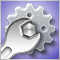Debugging MQL5 Programs
This article is intended primarily for the programmers who have already learned the language but have not fully mastered the program development yet. It reveals some debugging techniques and presents a combined experience of the author and many other programmers.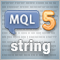MQL5 Programming Basics: Strings
The article covers everything you can do with strings in MQL5. It should be of interest primarily to novice MQL5 programmers, while experienced developers will have a good opportunity to summarize and systematize their knowledge.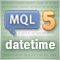MQL5 Programming Basics: Time
The article focuses on standard MQL5 functions for working with time, as well as programming techniques and practically useful functions for working with time that are required when creating Expert Advisors and indicators. Particular attention is paid to the general theory of time measurement. This article should be of interest primarily to novice MQL5 programmers.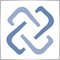MQL5 Cloud Network: Are You Still Calculating?
It will soon be a year and a half since the MQL5 Cloud Network has been launched. This leading edge event ushered in a new era of algorithmic trading - now with a couple of clicks, traders can have hundreds and thousands of computing cores at their disposal for the optimization of their trading strategies.